SBOA296 April   2021

Design Goals

InputOutput

Frequency

Supply
ViMaxVoMax

fMax

VccVee
9Vpp4.5Vpp

50kHz

5V0V

Design Description

This single-supply precision full-wave rectifier is optimized for high-input voltages. When Vi > 0V, D1 is reverse biased and the top part of the circuit, U1, is activated resulting in a circuit with a gain of 1V/V. When Vi < 0V, D1 is forward biased and the bottom part of the circuit, U2, is activated resulting in an inverting amplifier circuit with a gain of –1V/V.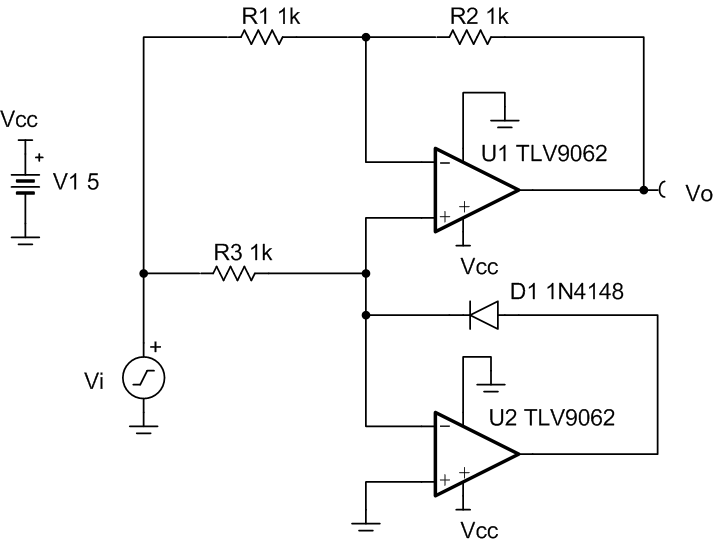Design Notes

1. Observe common-mode and output swing limitations of op amps.
2. R3 should be sized small enough that the leakage current from D1 does not cause errors for positive input cycles while ensuring the op amp can drive the load.
3. Use a fast switching diode for D1.
4. Resistor tolerance determines the gain error of the circuit.
5. Use a negative charge pump (such as the LM7705) for output swing requirements to GND to maintain linearity for output signals near 0V. For additional information. see Single-supply, low-input voltage, full-wave rectifier circuit.

Design Steps

1. Circuit analysis for positive input signals. D1 is reverse-biased disconnecting the output of U2 from the non-inverting input of U1.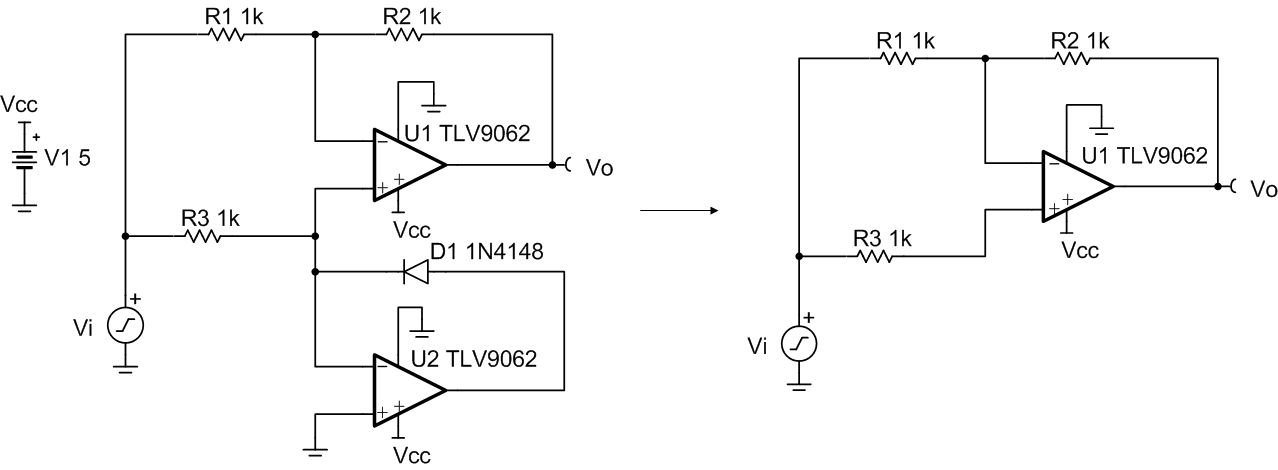Equation 1. $\frac{{V}_{o}}{{V}_{i}}=\left(-\frac{{R}_{2}}{{R}_{1}}\right)+\left(1+\frac{{R}_{2}}{{R}_{1}}\right)=1$
Equation 1. ${V}_{o}={V}_{i}$
2. Circuit analysis for negative input signals. D1 is forward biased, which connects the output of U2 to the non-inverting input of U1, which is GND.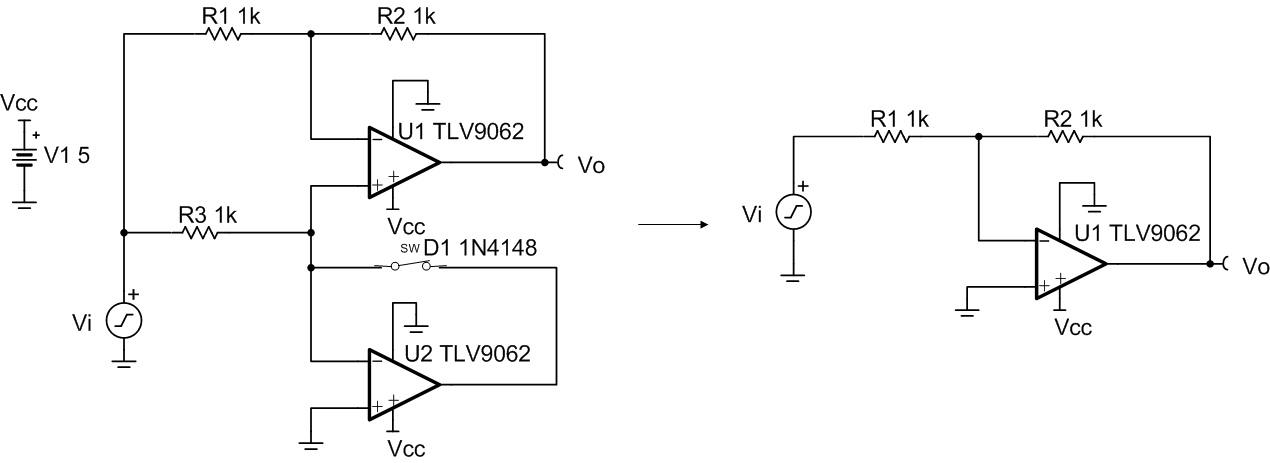Equation 1. $\frac{{V}_{o}}{{V}_{i}}=\left(-\frac{{R}_{2}}{{R}_{1}}\right)=-1$
Equation 1. ${V}_{o}=-{V}_{i}$
3. Select R1, R2, and R3.
Equation 1. $\frac{{V}_{o}}{{V}_{i}}=-\frac{{R}_{2}}{{R}_{1}}$

Equation 1.
Equation 1.

Design Simulations

Transient Simulation Results

A 1-kHz, 9-Vpp sine wave yields a 4.5-Vpp output sine wave.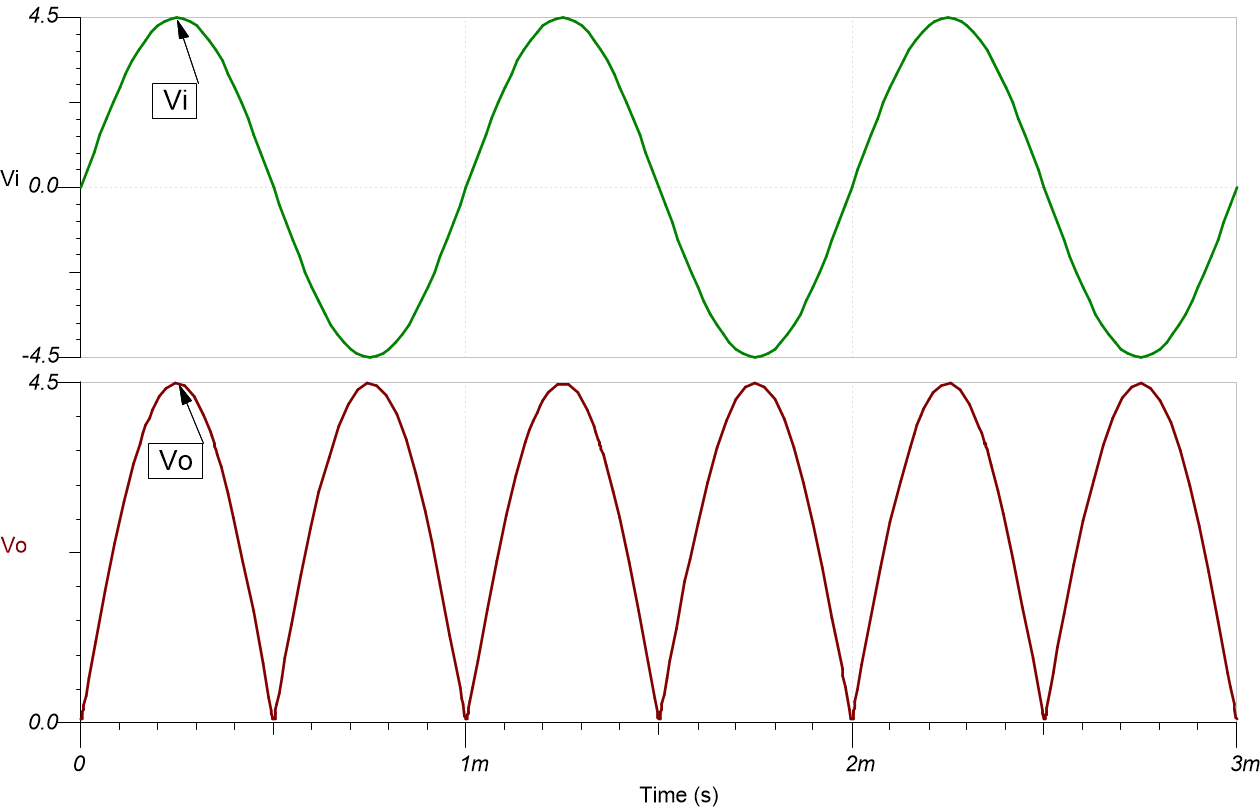A 50-kHz, 9-Vpp sine wave yields a 4.5-Vpp output sine wave.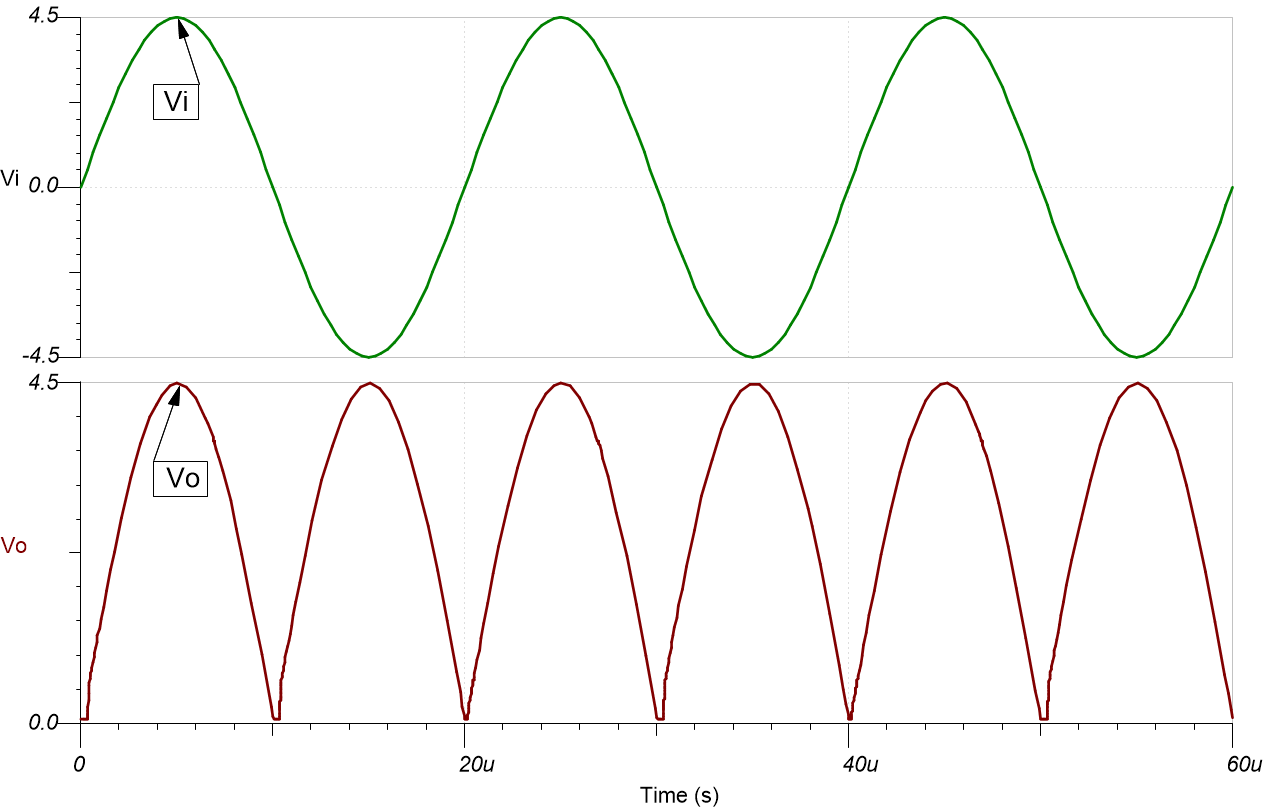Design References

1. See Analog Engineer's Circuit Cookbooks for the comprehensive TI circuit library.
2. SPICE Simulation File SBOC529.
3. TI Precision Labs
4. See the Single-Supply Low-Input Voltage Optimized Precision Full-Wave Rectifier Reference Design.

Design Featured Op Amp

TLV9062
Vss1.8V to 5.5V
VinCMRail–to–rail
VoutRail–to–rail
Vos0.30mV
Iq538µA
Ib0.5pA
UGBW10MHz
SR6.5V/µs
#Channels1, 2, 4
www.ti.com/product/TLV9062

Design Alternate Op Amps

OPA322OPA350
Vss1.8V to 5.5V2.7V to 5.5V
VinCMRail–to–railRail–to–rail
VoutRail–to–railRail–to–rail
Vos2mV0.15mV
Iq1.9mA5.2mA
Ib10pA0.5pA
UGBW20MHz38MHz
SR10V/µs22V/µs
#Channels1, 2, 41, 2, 4
www.ti.com/product/OPA322www.ti.com/product/OPA350